### Home > CAAC > Chapter 2 > Lesson 2.2.3 > Problem2-113

2-113.
1. The graph below shows distances traveled by Car A and Car B. Car A is represented by the line containing point A, and Car B is represented by the line containing point B. Use the graph to answer the following questions. Homework Help ✎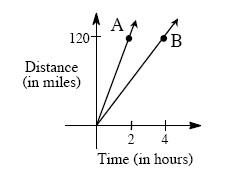1. Which car is traveling faster? How can you tell?

2. Find the coordinates of point A and point B.

3. How fast did Car A travel (in miles per hour)? How fast did Car B travel?

4. Does the distance Car A has traveled vary directly with the time? Why or why not?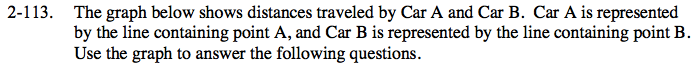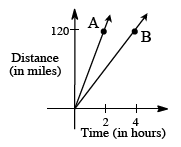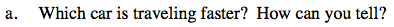The faster car has a steeper line.

Car A is traveling faster.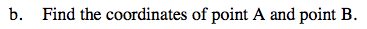A: (2, 120)
B: (4, 120)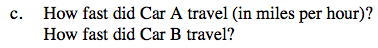$\text{Car A:}\frac{120\text{ miles}}{20\text{ hours}}$

Use the same process for Car B as you did for Car A.

Car A: 60 miles per hour

Car B: 30 miles per hour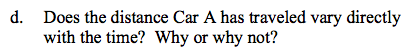Yes because the distance = constant · time.